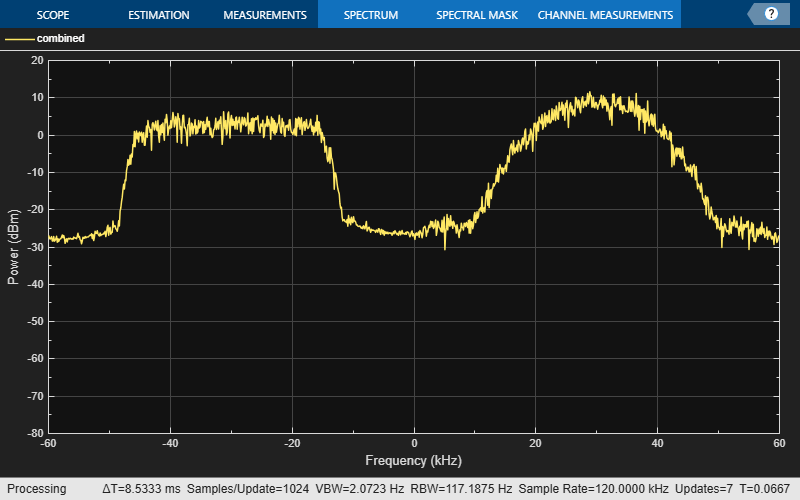# comm.MultibandCombiner

Frequency-shift and combine signals

## Description

The `comm.MultibandCombiner` System object™ interpolates, shifts input signals to the specified frequency bands, and then combines them into a single signal. For more information, see the Algorithms section.

To frequency-shift and combine signals:

1. Create the `comm.MultibandCombiner` object and set its properties.

2. Call the object with arguments, as if it were a function.

## Creation

### Syntax

``multibandcombiner = comm.MultibandCombiner``
``multibandcombiner = comm.MultibandCombiner(Name,Value)``

### Description

````multibandcombiner = comm.MultibandCombiner` creates a multiband combiner System object to frequency-shift and combine input signals.```

example

````multibandcombiner = comm.MultibandCombiner(Name,Value)` sets properties using one or more name-value arguments. For example, `'InputSampleRate',2e6` specifies an input signal sample rate of 2 MHz.```

## Properties

expand all

Unless otherwise indicated, properties are nontunable, which means you cannot change their values after calling the object. Objects lock when you call them, and the `release` function unlocks them.

If a property is tunable, you can change its value at any time.

Input signal sample rate in Hz, specified as a positive scalar.

Data Types: `double`

Frequency offsets in Hz, specified as one of these options.

• Scalar — Each channel of the input signal is frequency-shifted by this scalar value.

• 1-by- Nchan vector — Each channel of the input signal is frequency-shifted by the corresponding value in this vector. Nchan is the number of channels in the input signal `x`.

Data Types: `double`

Source of the output sample rate, specified as one of these values.

• `'Auto'` — The object interpolates the input signals to ensure that the resulting sample rate of the signals is sufficient to avoid distorting the frequency content of the original signals after they are frequency-shifted to produce the output signal.

• `'Property'` — Specify the output sample rate by using the `OutputSampleRate` property.

Data Types: `char` | `string`

Output signal sample rate in Hz, specified as a positive scalar.

#### Tips

To avoid distortion, specify this value to be greater than or equal to the automatically computed output sample rate. To determine the automatically computed output sample rate, first run the object with the `OutputSampleRateSource` property set to `'Auto'`.

#### Dependencies

To enable this property, set the `OutputSampleRateSource` property to `'Property'`.

Data Types: `double`

## Usage

### Syntax

``y = multibandcombiner(x)``

### Description

example

````y = multibandcombiner(x)` interpolates, frequency-shifts, and combines the input signal into one output signal.```

### Input Arguments

expand all

Input signals, specified as an Nsamp-by-Nchan matrix. Nsamp is the number of input samples per channel, and Nchan is the number of channels.

Data Types: `double` | `single`
Complex Number Support: Yes

### Output Arguments

expand all

Output signal, returned as an Nout-by-1 vector of the same data type as input signal `x`. Nout is the number of output samples. For more information, see Algorithms.

## Object Functions

To use an object function, specify the System object as the first input argument. For example, to release system resources of a System object named `obj`, use this syntax:

`release(obj)`

expand all

 `info` Characteristic information about multiband combining
 `step` Run System object algorithm `release` Release resources and allow changes to System object property values and input characteristics `reset` Reset internal states of System object

## Examples

collapse all

Combine two 60 KHz frequency bands that are adjacent to each other.

Set simulation parameters.

```M = 4; % QPSK modulation N = 2000; % Frame length Fs1 = 60e3; % Input sample rate```

Generate QPSK and GMSK signals.

```data = randi([0,M-1],N,1); modSig = pskmod(data,M,pi/4,'gray'); qpskTxFilter = comm.RaisedCosineTransmitFilter( ... OutputSamplesPerSymbol=2); qpsksig = qpskTxFilter(modSig); data = randi([0 1],N,1); gmskMod = comm.GMSKModulator( ... BitInput=true, ... SamplesPerSymbol=2); gmsksig = gmskMod(data);```

Create a multiband combiner System object. Use the `info` object function to determine the output sample rate for the combined signal. Use this output sample rate when configuring the spectrum analyzer objects.

```mbc = comm.MultibandCombiner( ... InputSampleRate=Fs1, ... FrequencyOffsets=[-30e3 30e3], ... OutputSampleRateSource='Auto'); mbcInfo = info(mbc); Fs2 = mbcInfo.OutputSampleRate```
```Fs2 = 120000 ```

Use the multiband combiner object to interpolate, frequency-shift, and combine the two signals.

`combinedsig = mbc([qpsksig,gmsksig]);`

Create spectrum analyzer System objects. Use the spectrum analyzer objects to view the individual signals at 60 kHz and the combined signal at 120 kHz.

```sa = dsp.SpectrumAnalyzer( ... NumInputPorts=2, ... SampleRate=Fs1, ... Method='Filter bank', ... AveragingMethod='Exponential', ... ShowLegend=true, ... ChannelNames={'qpsk','gmsk'}); sa(qpsksig,gmsksig); sacombined = dsp.SpectrumAnalyzer( ... SampleRate=Fs2, ... Method='Filter bank', ... AveragingMethod='Exponential', ... ShowLegend=true, ... ChannelNames={'combined'}); sacombined(combinedsig); release(sa)````release(sacombined)`expand all

## Version History

Introduced in R2021b